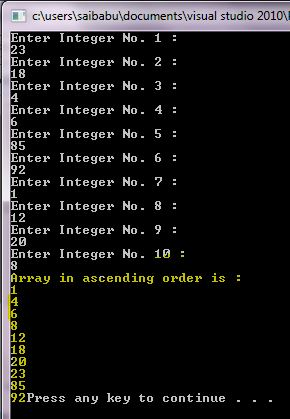# Answered! I do not understand what I need to do on this assignment. It states: View the code in the fileLoopingArrays.cpp…

I do not understand what I need to do on this assignment. It states: View the code in the fileLoopingArrays.cpp (in the Course Information area). Although the program compiles and runs, notice how difficult it is to read the code itself. Part of programming is making your code readable so other developers can read and maintain it. I am still learning but I don’t understand why this code is messy.

// LoopingArrays.cpp : This program loops through to create an array based on user input and then
// sorts the array in order of smallest integer to largest. Format the code following the code
// styling document.
//

Don't use plagiarized sources. Get Your Custom Essay on
Answered! I do not understand what I need to do on this assignment. It states: View the code in the fileLoopingArrays.cpp…
GET AN ESSAY WRITTEN FOR YOU FROM AS LOW AS \$13/PAGE

#include “stdafx.h”
#include <iostream>
#include <cstdlib>
using namespace std;

void main()
{
int array, t;
for (int x = 0; x<10; x++) {
cout << “Enter Integer No. ” << x + 1 << ” : ” << endl;
cin >> array[x];
}
for (int x = 0; x<10; x++) {
for (int y = 0; y<9; y++) {
if (array[y]>array[y + 1]) {
t = array[y];
array[y] = array[y + 1];
array[y + 1] = t;
}
}
}
cout << “Array in ascending order is : “;
for (int x = 0; x<10; x++)
cout << endl << array[x];
return;
}

Updated Code:

/*   C++ Program loops through to create an array based on user input
and then sorts the array in order of smallest integer to largest. */

#include “stdafx.h”
#include <iostream>
#include <cstdlib>

using namespace std;

//Main function
void main()
{
//Declaring integer array of size 10
int array, t;

//Reading 10 integer values from user
for (int x = 0; x<10; x++) {

//Prompting user to enter a value
cout << “Enter Integer No. ” << x + 1 << ” : ” << endl;

//Storing value in the array at corresponding index
cin >> array[x];
}

//Sorting logic

//Outer loop itertes for 10 times (Based on array size)
for (int x = 0; x<10; x++) {

//Inner loop iterates over each element in the array
for (int y = 0; y<9; y++) {

//Comparing side by side elements
if (array[y]>array[y + 1]) {

//Swapping elements
t = array[y];
array[y] = array[y + 1];
array[y + 1] = t;

} //If loop closing

}// Inner For Loop Closing

}// Outer For Loop Closing

cout << “Array in ascending order is : “;

//Iterating over array
for (int x = 0; x<10; x++)
{
//Printing array element one by one
cout << endl << array[x];
}

//Pausing the screen for a while
system(“pause”);

return;
}

______________________________________________________________________________________________

Sample Output: